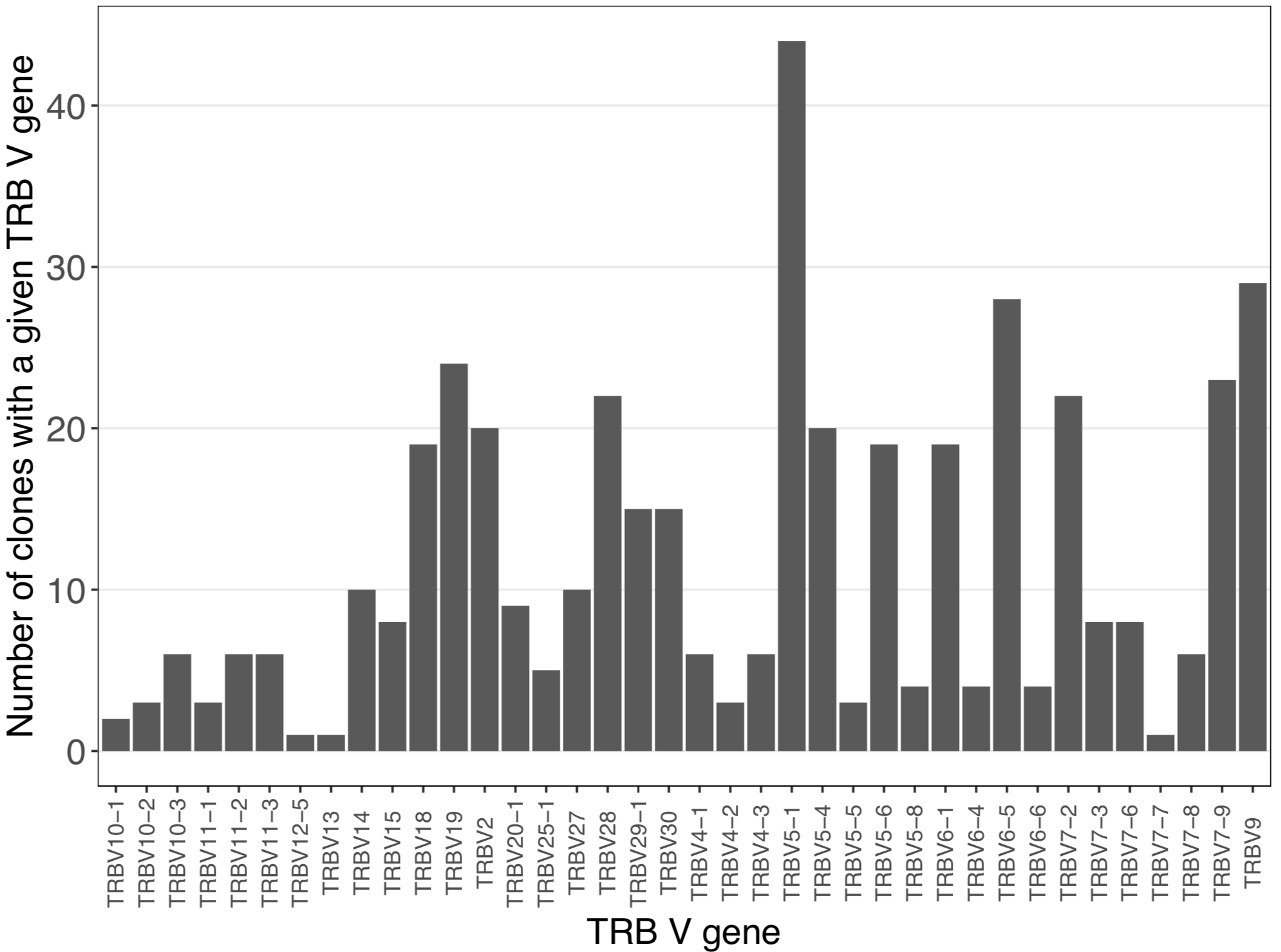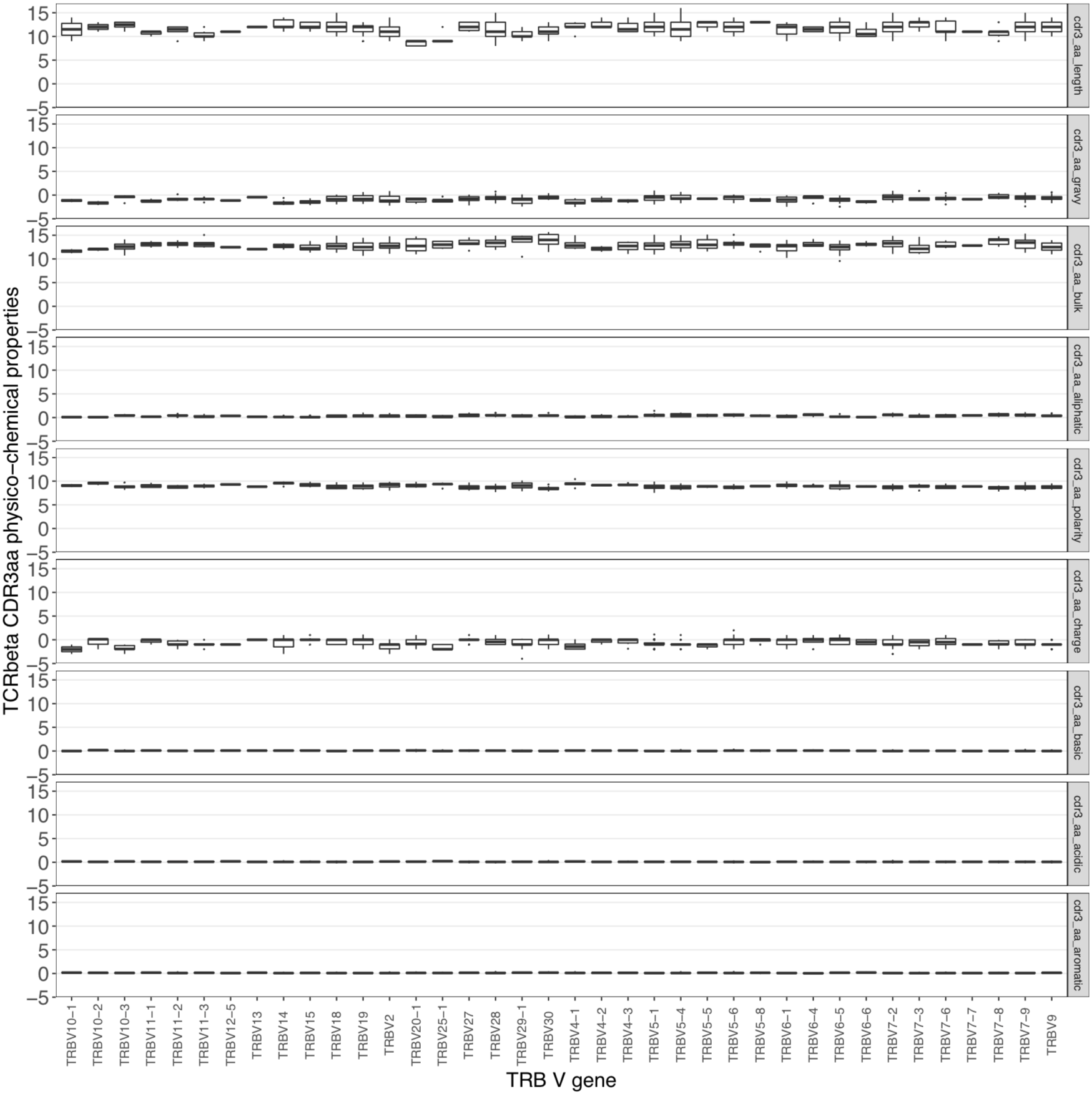# Integration use case: post-analysis of sequences with Immcantation¶

This use case will show how to perform a post-analysis of CMV associated TCRβ sequences using Immcantation/ These sequences were identified through the method published by Emerson et al., which was reproduced inside immuneML. The analysis used to obtain these sequences is described in Manuscript use case 1: Reproduction of a published study inside immuneML, where the sequences were exported using the RelevantSequenceExporter.

Download the sequence file here: relevant_sequences.csv

This analysis requires the R packages alakazam and reshape2 to be installed.

library(alakazam) #https://alakazam.readthedocs.io/en/stable/install/
#library(dplyr)
library(reshape2)

# Read in of CMV-associated sequences
relevant_sequences <- read.csv("relevant_sequences.csv") # Column names are AIRR-compliant
relevant_sequences = relevant_sequences[!(relevant_sequences\$v_call==""), ] # Remove sequences with missing V gene annotation
colnames(relevant_sequences) <- c("v_genes") #renaming of column in order for countGenes function to work properly

# V gene analysis using Immcantation
gene <- countGenes(relevant_sequences, gene="v_genes", mode="gene")

ggplot(as.data.frame(gene), aes(x=gene, y=seq_count)) +
geom_bar(stat = "identity") +
labs (x = "TRB V gene", y = "Number of clones with a given TRB V gene") +
theme_bw() +
theme(axis.text.y = element_text(size= 16),
axis.text.x = element_text(vjust = 0.5, hjust =0.5, size = 10, angle = 90),
axis.title.y = element_text(vjust=1, size = 16),
axis.title.x = element_text(size= 16,  vjust=0.5),
panel.grid.major.x = element_blank(),
panel.grid.minor.y = element_blank(),
panel.border = element_rect(colour = "black"))

# Analyis of TCRB-amino acid physico-chemical properties using Immcantation
db_props <- aminoAcidProperties(relevant_sequences, seq="sequence_aa", nt=FALSE, trim=TRUE,label="cdr3")
db_props_melt_df <- melt(db_props)

ggplot(db_props_melt_df, aes(x=v_genes, y=value)) +
labs (x = "TRB V gene", y = "TCRbeta CDR3aa physico-chemical properties") +
facet_grid(variable~.) +
geom_boxplot(outlier.size = 0.1) +
theme_bw() +
theme(axis.text.y = element_text(size= 16),
axis.text.x = element_text(vjust = 0.5, hjust =0.5, size = 10, angle = 90),
axis.title.y = element_text(vjust=1, size = 16),
axis.title.x = element_text(size= 16,  vjust=0.5),
panel.grid.major.x = element_blank(),
panel.grid.minor.y = element_blank(),
panel.border = element_rect(colour = "black"))


The alakazam (Immcantation) countGenes() function provides the insight that TRBV5-1 is the most used V gene among the CMV-associated TCRβ sequences:The aminoAcidProperties() function enables insight into the variation of PC properties across those V genes used by the CMV-associated TCRβ sequences: